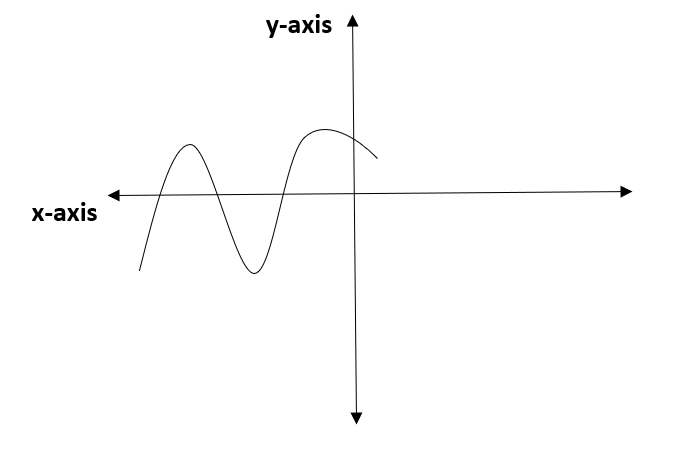The graph of y=p(x)   is given in the following figure, for some polynomial p x .   Find the number of zeroes of p(x)  .

# The graph of   is given in the following figure, for some polynomial   Find the number of zeroes of  .1. A

2. B

3. C

4. D

Fill Out the Form for Expert Academic Guidance!l

+91

Live ClassesBooksTest SeriesSelf Learning

Verify OTP Code (required)

### Solution:

We have been given a graph of   for some polynomial  . We need to determine the zeroes of  .
The number of zeroes in a polynomial is determined by the number of times the graph cuts the x-axis.
Given graph is,From the graph we can observe that the number of times at which the graph cuts the x -axis is
Hence, the number of zeroes for the polynomial   is
Therefore, option 3 is correct.

## Related content

 Area of Square Area of Isosceles Triangle Pythagoras Theorem Triangle Formula Perimeter of Triangle Formula Area Formulae Volume of Cone Formula Matrices and Determinants_mathematics Critical Points Solved Examples Type of relations_mathematics+91

Live ClassesBooksTest SeriesSelf Learning

Verify OTP Code (required)